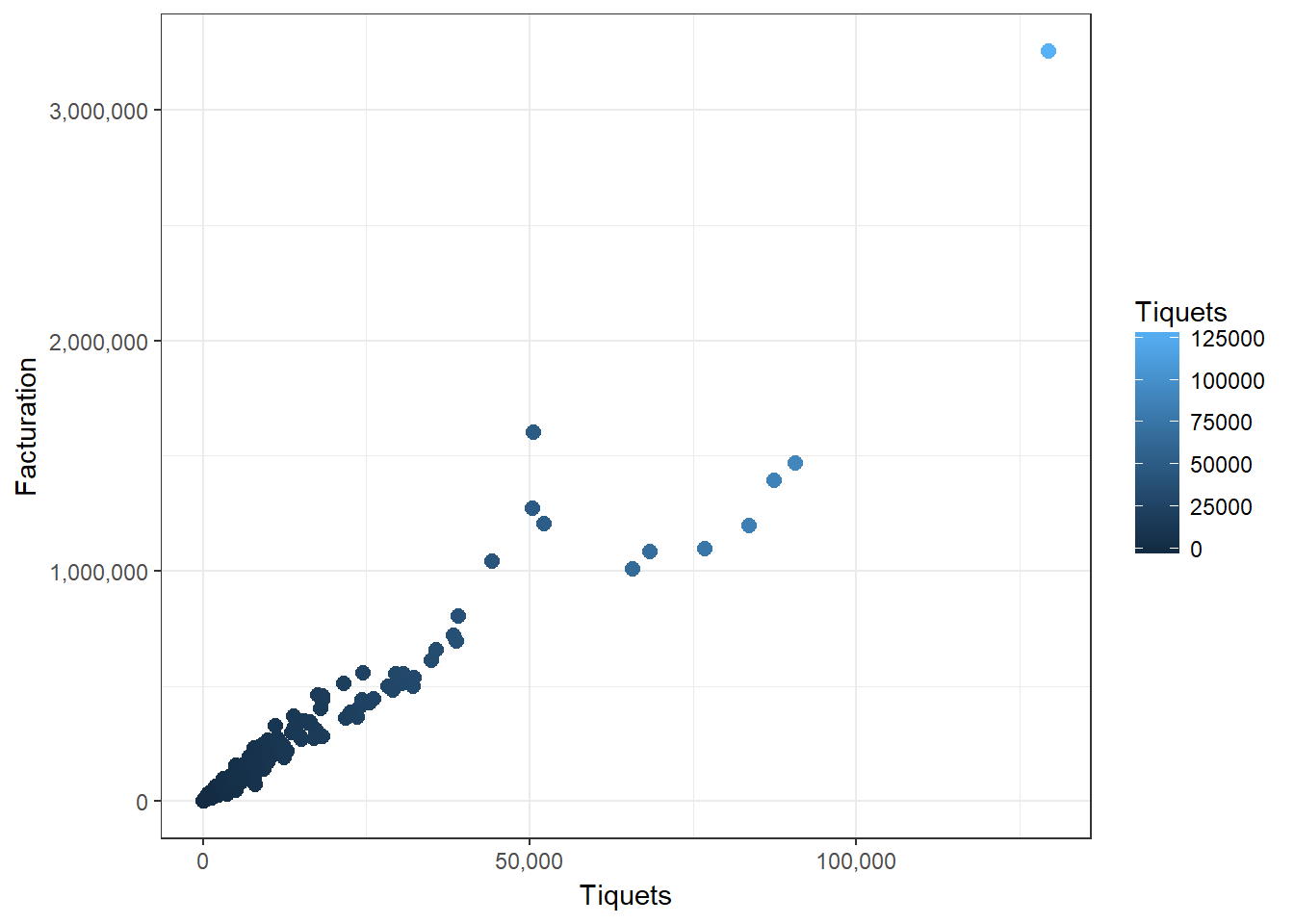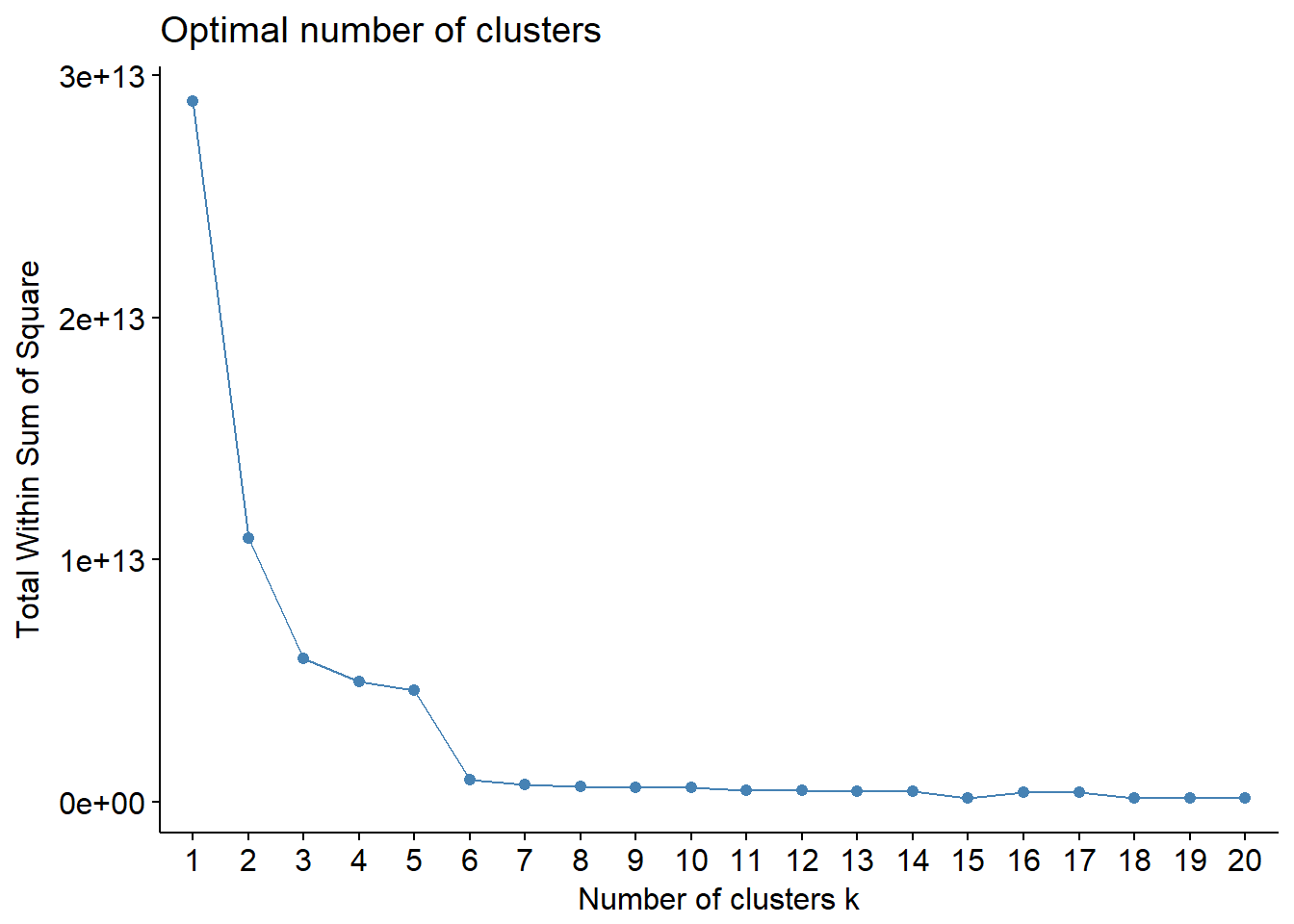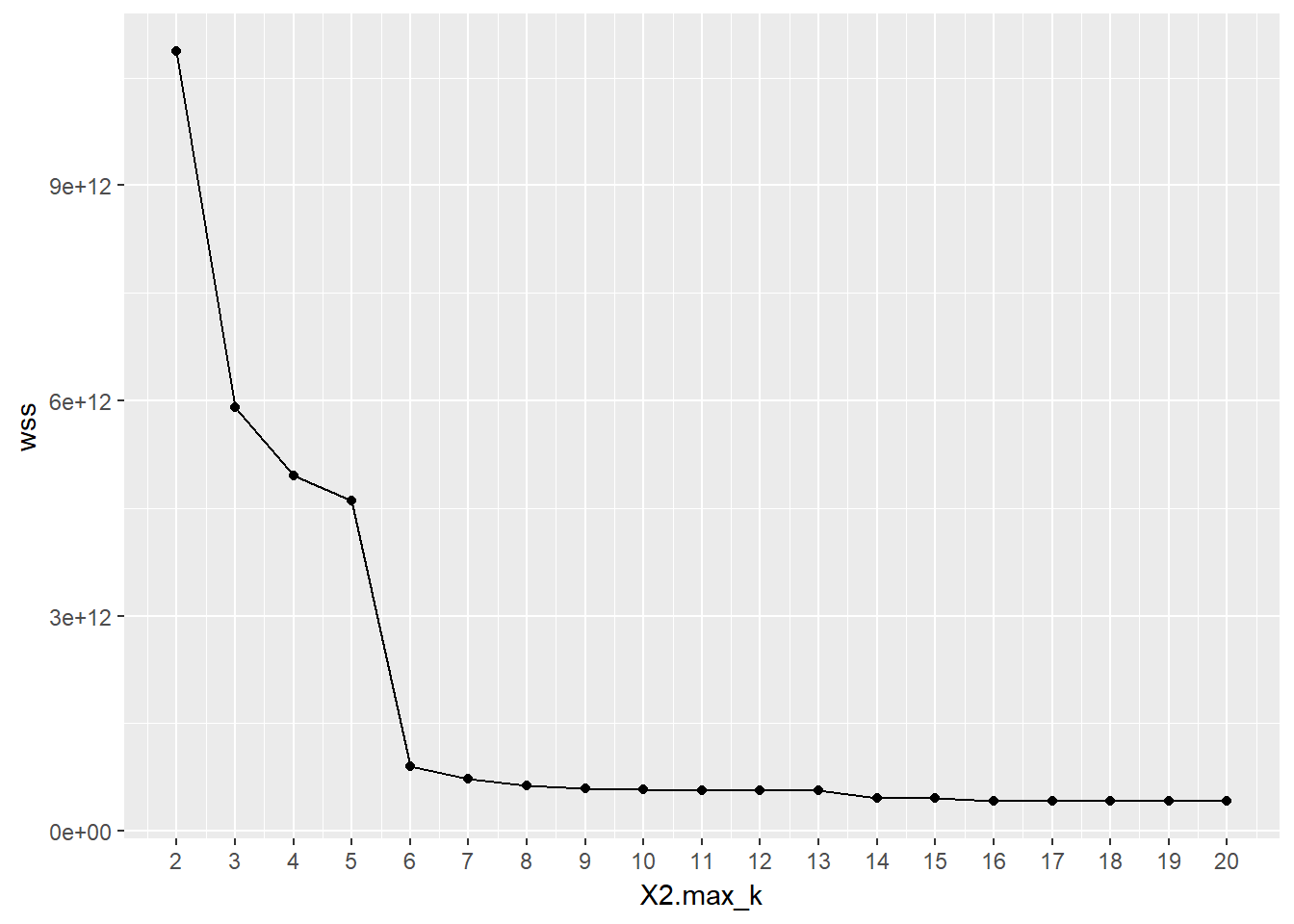﻿ Example of k-means in R - Blog R

# Example of k-means in R

## K-means

Hi everyone, today we go to explain how to do a cluster segmentation, the term clustering refers to a wide range of unsupervised techniques whose purpose is to find patterns or groups (clusters) within a set of observations.

In the world of cluster we have three kinds of cluster but for this case we explain you the Partitioning Clustering, this type of algorithm requires the user to specify in advance the number of clusters that will be created (for example K-means).

For this example we will use a dataset with information of different shops and the libraries:

# Load the libraries
library(dplyr)
library(magrittr)
library(animation)
library(ggplot2)
library(factoextra)

We create the new data set with the data we want use.

ShopsData <- read.csv("ShopsData.csv")

We can observe the data, using these two functions:

str(ShopsData)
## 'data.frame':    346 obs. of  3 variables:
##  $Shop : int 2 4 5 7 8 9 10 19 29 34 ... ##$ Tiquets    : int  17504 2216 6585 9088 1076 5106 1370 4233 11150 50390 ...
##  $Facturation: num 461726 60023 162173 242707 28712 ... summary(ShopsData) ## Shop Tiquets Facturation ## Min. : 2.00 Min. : 0 Min. : 0 ## 1st Qu.:38.00 1st Qu.: 1475 1st Qu.: 29064 ## Median :58.00 Median : 4280 Median : 76033 ## Mean :55.66 Mean : 8716 Mean : 164436 ## 3rd Qu.:78.00 3rd Qu.: 8778 3rd Qu.: 162773 ## Max. :94.00 Max. :129412 Max. :3256098 ## NA's :7 # If the data have values very different between variables, we can use the function scale, but in this case we don�t need it #ShopsData <- as.data.frame(scale(ShopsData)) As we can see in the chart, we have different shops with tickets and the total of money, the majority of shop don�t have to many tickets or facturation, our objective is create a clusters to be able to classify the stores. ggplot(data = ShopsData, aes(x = Tiquets, y = Facturation, color = Tiquets)) + geom_point(size = 2.5) + scale_x_continuous(labels = scales::comma)+ scale_y_continuous(labels = scales::comma)+ theme_bw()Now, one of the most important problems is know how many clusters we will need for a correct segmentation, one technique to choose the best k is called the elbow method. This method uses within-group homogeneity or within-group heterogeneity to evaluate the variability. In other words, you are interested in the percentage of the variance explained by each cluster. here we have two options: 1� Using the function fviz_nbclust, from the �factoextra� library: fviz_nbclust(x = ShopsData[2:3], FUNcluster = kmeans, method = "wss", k.max = 20, diss = get_dist(ShopsData[2:3], method = "euclidean"), nstart = 50)In this case we can see that the best value for k is 6 because from curve 6 the curve is stabilized. The other option is create a function with sapply: kmean_knumbers <- function(k) { cluster <- kmeans(ShopsData[2:3], k) return (cluster$tot.withinss)
}
# Set maximum cluster
max_k <-20
# Run algorithm over a range of k
wss <- sapply(2:max_k, kmean_knumbers)
# Create a data frame to plot the graph
elbow <-data.frame(2:max_k, wss)

# Plot the graph with gglop
ggplot(elbow, aes(x = X2.max_k, y = wss)) +
geom_point() +
geom_line() +
scale_x_continuous(breaks = seq(1, 20, by = 1))After see that the better value for k is 6, we will to proceed to create the clusters.

Now we have the data and the number of k, so we can create the next function:

km_clusters <- kmeans(x = ShopsData[2:3], centers = 6, nstart = 50)

If we want know the number of cluster for each shop, we can do that passing the list to the dataframe.

ShopsData$cluster <- km_clusters$cluster

ShopsData$cluster <- as.character(ShopsData$cluster)

ggplot(data = ShopsData, aes(x = Tiquets, y = Facturation, color = cluster)) +
geom_point(size = 2.5) +
scale_x_continuous(labels = scales::comma)+
scale_y_continuous(labels = scales::comma)+
theme_bw()

Finally, we store each cluster in a dataframe to be able to analyze them separately.

NumberOfCluster <- as.factor(ShopsData$cluster) AverageDataCluster <- data.frame() for (i in levels(NumberOfCluster)) { x <- subset(ShopsData, ShopsData$cluster == i)
assign( paste("Cluster", i, sep = " "), x )
Name <- paste("Cluster", i, sep = " ")
AverageTickets <- sum(mean(x$Tiquets)) AverageFact <- sum(mean(x$Facturation))
NewData <- data.frame("Cluster" = Name, "Average Tickets" = AverageTickets, "Average Fact" = AverageFact)
AverageDataCluster <- rbind(AverageDataCluster,NewData)

}

I hope it has helped you in your segmentations, any doubt leave it in the comments

SummaryArticle Name
Example of k-means in R
Description
Here one example with k-means in R, if you need to create a segmentation and visualizate it you can do it with your data this is a powerful tool .
Author
Publisher Name
Blog-R
Publisher Logo